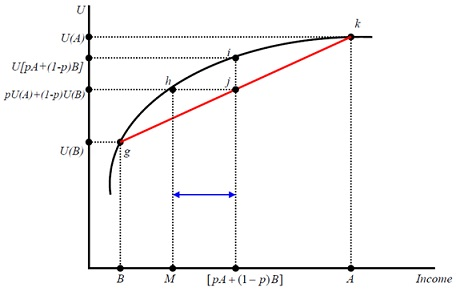#### von Neumann-Morgenstern Utility Function

The von Neumann-Morgenstern Utility Function:

A lottery L will pay \$A with likelihood p and \$B with (1− p). If we can assign numbers (cardinal utilities) U(A) and U(B) then the expected utility of this lottery L is able to be defined as follows:

U(L) = pU( A) + (1− p)U(B)

Beside assigning two (hypothetical) extreme numbers A, B, U(A) and U(B) respectively. Assume that A = \$100,000 and B = − \$3,000.

As well U( A) = 100 and U(B) = 0

Using the continuity axiom we are able to calculate the specific probability p that can make this decision-maker indifferent among buying this lottery (risky asset) as well as having any specific amount of money (safe asset). Let’s take \$25000 as safe asset. If this person says that his p equals 0.4 then the utility that \$25000 will create:

U(\$25,000) = 0.4 ×U(\$100000) + (1− 0.4) ×U(−3000) = 0.4 ×100 + 0.6 × 0 = 40

Using this instance the expected value of the award from this lottery is calculated simply:

\$[pA + (1− p)B].

The risk averse person will favour the situation of having the expected value amount for sure to playing this lottery. In his point of view the expected utility of lottery is smaller than the utility of expected value.

pU(A) + (1− p)U(B) <U[ pA + (1− p)B]

This characteristic is analogous to the mathematical implication of strictly concave function, which denotes that risk averse person will have (strictly) concave utility curve.

Concave Function:

Presume there is a function y = f (x). Take any two values of x1, x2. And let x3 be located in between x1 and x2 . x3 can be mathematically expressed as follows:

x3 = kx1 + (1− k)x2 , 0 < k < 1

Hence, x3 is a convex combination of x1 and x2. If k is close to 0, x3 approaches to x2. Concave function can be expressed as:

kf (x1) + (1− k) f (x2) < f [kx1 + (1− k)x2]

Likewise, convex function can be expressed as:

kf (x1) + (1− k) f (x2) > f [kx1 + (1− k)x2]